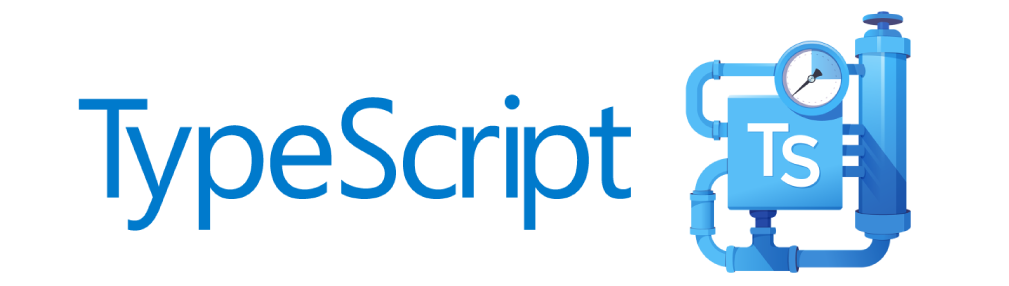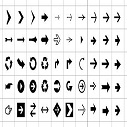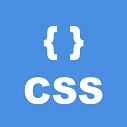# Python Conditions and If statements

Python supports the usual logical conditions from mathematics:

• Equals: `a == b`
• Not Equals: `a != b`
• Less than:` a < b`
• Less than or equal to: `a <= b`
• Greater than: `a > b`
• Greater than or equal to: `a >= b`

These conditions can be used in several ways, most commonly in "if statements" and loops.

An "if statement" is written by using the `if` keyword.

```a = 33
b = 200
if b > a:
print("b is greater than a")```

### Indentation

Python relies on indentation, using whitespace, to define scope in the code. Other programming languages often use curly-brackets for this purpose.

If statement, without indentation (will raise an error):
```a = 33
b = 200
if b > a:
print("b is greater than a") # you will get an error```

### Elif

The `else` keyword catches anything which isn't caught by the preceding conditions.

```a = 200
b = 33
if b > a:
print("b is greater than a")
elif a == b:
print("a and b are equal")
else:
print("a is greater than b")```

You can also have an `else` without the `elif`:

```a = 200
b = 33
if b > a:
print("b is greater than a")
else:
print("b is not greater than a")```

### Short Hand If

If you have only one statement to execute, you can put it on the same line as the if statement.

`if a > b: print("a is greater than b")`

### Short Hand If ... Else

If you have only one statement to execute, one for if, and one for else, you can put it all on the same line:

`print("A") if a > b else print("B")`

You can also have multiple else statements on the same line:

`print("A") if a > b else print("=") if a == b else print("B")`

### And

The `and` keyword is a logical operator, and is used to combine conditional statements:

```if a > b and c > a:
print("Both conditions are True")```

### Or

The `or` keyword is a logical operator, and is used to combine conditional statements:

```if a > b or a > c:
print("At least one of the conditions is True")```Uk01 on Apr 29, 2015 at 12:04 am

If you like dEexams.com and would like to contribute, you can write your article here or mail your article to admin@deexams.com . See your article appearing on the dEexams.com main page and help others to learn.

#### Comments( 0)

##### WEB TECHNOLOGY##### TypeScript Programmning Language##### New HTML5 APIs##### HTML Arrow -> Range: Decimal 8592-8703. Hex 2190-21FF.##### How To Override One CSS Class With Another×

## Forgot Password

Please enter your email address below and we will send you information to change your password.